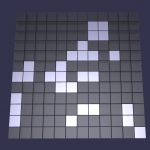# Cellular Automata Algorithms (Part 1)

There are various algorithms to describe the development of cellular systems and how they maintain, develop or degrade overtime. It is usually represented by a grid system where the various cells positions are identified and their state is stored as a variable. The state of the cell and its neighboring cells will determine how the next step in the algorithm is calculated.

## Conway’s LifeThe simplest of these algorithms is known as Conway’s Life, in which the cells are in one of two states: alive or dead. The initial set up is determined by the user, but once in run time this is a player less game meaning that a set of rules dictates the outcome without any additional input. The rules are simple, taking into account the current cells and its “neighbors” states. A neighbor is a cell touching the current cell in any direction horizontal, vertical, and diagonal, this whole process can additionally be extended into 3 dimensions.

### Rules:

1 – Any living cell (state 1) with fewer then two live neighbors dies (state 0).
2 – Any living cell (state 1) with two or three live neighbors does not change state.
3 – Any living cell (state 1) with more then three live neighbors dies (state 0).
4 – Any dead cell (state 0) with exactly three live neighbors becomes a live cell (state 1).

The position of the living cells set up by the user, is considered the seed of the system. Each generation or tick is calculated with the births and deaths occurring simultaneously, with the rules being applied to each generation to create the next.

Here is a simple webGL example: https://www.babylonjs-playground.com/frame.html#TWRM2D#29
You can slide the bar on the left out to see the code.

## q-state Life

Taking the idea of Conway’s Life and developing it a little farther the cells in q-state may be in any q state, 1, 2, …, q. With colors representing each state, black always representing 1 and white always representing q.

### Rules:

1 – Select four integers k1, k2, k3 and k4 from 0 through 900 in value.
2 – For each cell S denotes the sum of the states of its neighbors (S excludes state of the cell itself).
3 – s denotes the state of the cell. If s > q/2 then if k1 <= S <= k2 then add 1 (if s < q) to the state of the cell, otherwise subtract 1 (if s > 1).
4 – Otherwise (if s <= q/2) if k3 <= S <= k4 then add 1 (if s < q) to the state of the cell, otherwise subtract 1 (if s > 1).

Sources:
https://hermetic.ch/pca/algorithms.htm